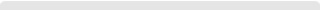���屡Ĥ�ṹ�񶯷��������-��Ŀ����-��ˮ�ؼӸ�-����Ĥ|Ĥ�Ӹ�-�����Ӹ�-Ĥ�ṹ��˾-�Ϻ���ϲĤ�ṹ�������޹�˾

#EN��ҳ > ���Ŷ�̬ > ��˾��̬

# ���屡Ĥ�ṹ�񶯷��������

## ����ʱ��:2021��3��19�� �����:2186

���Ϳռ䱡Ĥ�ṹ�����������ᡢ�ղ����С���ɱ��͡����۵����ŵ�õ��㷺��ע,Ȼ����������Ĥ�ṹ��ͨ����������,����Ĵ��ڲ����ή�ͱ�Ĥ�ṹ�ı��澫��,����ı䱡Ĥ�е�Ӧ���ֲ�,Ӱ�챡Ĥ�ṹ�Ķ�̬����[2,3]������������,KUKATHASAN et alͨ��ʵ������˲�ͬ������̬�±�Ĥ�����Եı仯,��֤������Ĵ��ڿɸı䱡Ĥ�ṹ�����ͺ͹���Ƶ��;HOSSAIN et al��ABAQUS���������뷣������������ģ��,˵������Ա�Ĥ�ṹ����������Ӱ�졣���,�Ա�Ĥ�ṹ���ж�̬���Է���ʱ,Ӧ�����������������������͸նȾ���ı仯��

������ANSYS����Ԫ�����в��ÿǵ�Ԫ���������α�Ĥ�ṹ,�������÷���������Ԫ���������õ���Ĥ�ṹ��������̬,����������Ϣ��������Ԫģ�ͽ���ģ̬����,�Ա���������2������±�Ĥ�ṹ���׹���Ƶ�ʺ�ģ̬���͡��Գ�ʼ������̬��Ϊ��ƽ��λ��,�ڱ�Ĥ����һ��ʩ�Ӵ�ֱ��Ĥ�淽��ĳ���Ŷ�����˲̬����[7,8],�õ����屡Ĥ�������ߡ��ڴ˻�����,���PID����ϵͳ,ͨ��MATLAB/ANSYS���Ϸ�����֤���Ʒ����������񶯵���Ч��,�Ӷ���߱�Ĥ�ṹ���ڹ��ȶ���[9,10]��

### 1 ���屡Ĥģ̬����

����ͼ1��ʾ������(�߳�L=500 mm)��Ĥ�ṹ������Ԫģ��,��Ĥ����ģ��E=2.5 GPa,���ɱ���=0.34,�ܶ���=1 400 kg/m3,���h=25 ��m��Ϊ����Ӧ�����С���ⲻ���������,��4�����г�,L1=25 mm���߽�����Ϊ�Ľǹ�֧,��4�����㴦��ֱ����ʩ���ط��߷����λ���غ�VX=1.0 mm,VY=0.25 mm��

���������о��ɹ�,��X�����Y����������ֵ���ڵ���4ʱ�γɹᴩ���ĵĺ�������,����ģ�Ͳ�����ģ̬����,ȥ���������ͺ���ȡǰ4�׹���Ƶ�ʺ�ģ̬����,��ͼ2��ʾ��������ͼ�ϼ��ɿ���������̬,˵������Ա�Ĥ�ṹ�Ķ�̬���Բ�����Ӱ�졣

### 2 ���屡Ĥ˲̬����

��Ĥ�ṹ������ʱ,������������,�񶯹��κ���Ϊwv,��������Ĵ���,����������κ���wz,���񶯹����б�Ĥ�����α�����Ep1Ϊ:��Ĥ��Ӧ������Ep2���Ա�ʾΪ:

$\begin{array}{l}E{}_{\text{p}2}=\frac{Eh{}_{m}}{2\left(1-\mathrm{��}{}^{2}\right)}\iint \left(\mathrm{��}{}_{x}^{2}+\mathrm{��}{}_{y}^{2}+\\ 2\mathrm{��}\mathrm{��}{}_{x}\mathrm{��}{}_{y}+\frac{1-\mathrm{��}}{2}\mathrm{��}{}_{xy}^{2}\right)\text{d}x\text{d}y\text{ }\text{ }\text{ }\text{ }\text{ }\text{ }\text{ }\text{ }\text{ }\left(2\right)\end{array}$

ʽ��:��x,��y�ֱ���X�����Y�����Ӧ��,��xy����Ӧ�䡣�������񶯹��κ�����������κ����Ĺ�ϵ���Ա�ʾΪ:��ʽ(3)���뵽ʽ(2),�õ���ĤӦ�����ܵı���ʽΪ:��Ĥ������ʱ�Ķ���Ϊ:

$E{}_{\text{v}}=\frac{1}{2}\mathrm{��}h{}_{m}\iint \left(\frac{\partial w{}_{\text{v}}}{\partial t}\right){}^{2}\text{d}x\text{d}y$ (5)

��wv��wz����ʾ�ɼ�����ʽ:��ʽ(6)�ֱ����ʽ(1)��(4),�õ��񶯹�����������Ϊ:

$\begin{array}{l}E{}_{\text{p}}=E{}_{\text{p}1}+E{}_{\text{p}2}=\\ \frac{1}{2}\left(k{}_{ijkl}q{}_{ijkl}+k{}_{ij}q{}_{ij}+{k}^{��}{}_{ijkl}\right)\text{ }\text{ }\text{ }\text{ }\text{ }\text{ }\text{ }\text{ }\text{ }\left(7\right)\end{array}$

��ʽ(6)����ʽ(5),�õ����ܵı���ʽ:

$E{}_{\text{v}}=\frac{1}{2}m{}_{ij}\stackrel{�B}{q}{}_{i}\stackrel{�B}{q}{}_{j}$ (8)

ʽ��:kijkl,kij,k��ijkl,mij�ľ������ʽ����:

$\begin{array}{l}k{}_{ijkl}=\frac{Eh{}_{m}}{4\left(1-\mathrm{��}{}^{2}\right)}\iint \left(\frac{\partial w{}_{\text{v}i}}{\partial x}\frac{\partial w{}_{\text{v}j}}{\partial x}\frac{\partial w{}_{\text{v}k}}{\partial x}\frac{\partial w{}_{\text{v}l}}{\partial x}+\\ \frac{\partial w{}_{\text{v}i}}{\partial y}\frac{\partial w{}_{\text{v}j}}{\partial y}\frac{\partial w{}_{\text{v}k}}{\partial y}\frac{\partial w{}_{\text{v}l}}{\partial y}+2\frac{\partial w{}_{\text{v}i}}{\partial x}\frac{\partial w{}_{\text{v}j}}{\partial x}\frac{\partial w{}_{\text{v}k}}{\partial y}\frac{\partial w{}_{\text{v}l}}{\partial y}\right)\text{d}x\text{d}y\\ k{}_{ij}=\text{��}\iint \left(\frac{\partial {}^{2}w{}_{\text{v}i}}{\partial x{}^{2}}\frac{\partial {}^{2}w{}_{\text{v}j}}{\partial x{}^{2}}+\frac{\partial {}^{2}w{}_{\text{v}i}}{\partial y{}^{2}}\frac{\partial {}^{2}w{}_{\text{v}j}}{\partial y{}^{2}}+\\ 2\mathrm{��}\frac{\partial {}^{2}w{}_{\text{v}i}}{\partial x{}^{2}}\frac{\partial {}^{2}w{}_{\text{v}j}}{\partial y{}^{2}}+2\left(1-\mathrm{��}\right)\frac{\partial {}^{2}w{}_{\text{v}i}}{\partial x\partial y}\frac{\partial {}^{2}w{}_{\text{v}j}}{\partial x\partial y}\right)\text{d}x\text{d}y-\\ \frac{Eh{}_{m}}{2\left(1-\mathrm{��}{}^{2}\right)}\iint \left(\frac{\partial w{}_{\text{v}i}}{\partial x}\frac{\partial w{}_{\text{v}j}}{\partial x}\frac{\partial w{}_{\text{z}i}}{\partial x}\frac{\partial w{}_{\text{z}j}}{\partial x}+\\ \frac{\partial w{}_{\text{v}i}}{\partial y}\frac{\partial w{}_{\text{v}j}}{\partial y}\frac{\partial w{}_{\text{z}i}}{\partial y}\frac{\partial w{}_{\text{z}j}}{\partial y}+\mathrm{��}\frac{\partial w{}_{\text{v}i}}{\partial x}\frac{\partial w{}_{\text{v}j}}{\partial x}\frac{\partial w{}_{\text{z}i}}{\partial y}\frac{\partial w{}_{\text{z}j}}{\partial y}+\\ \mathrm{��}\frac{\partial w{}_{\text{v}i}}{\partial y}\frac{\partial w{}_{\text{v}j}}{\partial y}\frac{\partial w{}_{\text{z}i}}{\partial x}\frac{\partial w{}_{\text{z}j}}{\partial x}+\\ 2\left(1-\mathrm{��}\right)\frac{\partial w{}_{\text{v}i}}{\partial x}\frac{\partial w{}_{\text{z}i}}{\partial x}\frac{\partial w{}_{\text{v}j}}{\partial y}\frac{\partial w{}_{\text{z}j}}{\partial y}\right)\text{d}x\text{d}y\\ {k}^{��}{}_{ijkl}=\frac{Eh{}_{m}}{4\left(1-\mathrm{��}{}^{2}\right)}\iint \left(\frac{\partial w{}_{\text{z}i}}{\partial x}\frac{\partial w{}_{\text{z}j}}{\partial x}\frac{\partial w{}_{\text{z}k}}{\partial x}\frac{\partial w{}_{\text{z}l}}{\partial x}+\\ \frac{\partial w{}_{\text{z}i}}{\partial y}\frac{\partial w{}_{\text{z}j}}{\partial y}\frac{\partial w{}_{\text{z}k}}{\partial y}\frac{\partial w{}_{\text{z}l}}{\partial y}+2\frac{\partial w{}_{\text{z}i}}{\partial x}\frac{\partial w{}_{\text{z}j}}{\partial x}\frac{\partial w{}_{\text{z}k}}{\partial y}\frac{\partial w{}_{\text{z}l}}{\partial y}\right)\text{d}x\text{d}y\\ m{}_{ij}=\mathrm{��}h{}_{m}\iint w{}_{\text{v}i}w{}_{\text{v}j}\text{d}x\text{d}y\end{array}$

��ʽ(7)-(8)�����������շ���,���յõ����屡Ĥ�ṹ�ķ�������΢�ַ���:��Ĥ���������ʱ,Ĥ���ϸ������ƽ��λ�ò�����0,������һ�ڽ���������Ԫģ��,��VX=1.0 mm,VY=0.25 mmʱ�õ�ͼ3��ʾ������̬,���������ֵ��Ϊ����ʱ��ƽ��λ������˲̬����,��1.3 sʱʩ�ӳ���Ŷ�,�Ŷ���ֵΪ4 mm,����ʱ��Ϊ0.02 s,������ʱ��Ϊ4 s���ں���/POST26����ȡ�Ŷ�ʩ�ӽڵ�ź������ֵP��ڵ�Ŷ�Ӧ����ֵ,ͨ��/OUTPUTָ�ʱ������ֵ���浽�ı��ĵ���,���Ƶõ�ͼ4��ʾ���Ŷ��㴦������ͼ,���Կ���,��1.3 s֮ǰ�õ㴦�ھ�ֹ״̬,1.3 sʱ�����Ŷ��ź�,֮��Ĥ�濪ʼ��,��Ƶ��ԼΪ28.5 Hz,��ͼ2�е�һ�׹���Ƶ��ֵ���Ǻϡ�

ͼ5Ϊ�����ֵP�㴦��������,1.3 sǰ�õ㴦��ʼƽ��λ��Ϊ���������0.80 mm��

### 3 MATLAB/ANSYS�����񶯿��Ʒ���

��ͼ6��ʾ,�ڱ�Ĥ�ṹ���㸽����װѹ��������,ͨ����ֱĤ����˶���Ч���Ʊ�Ĥ�񶯡����ڱ�Ĥ�ṹ��ģ���Ǹ߶���ϵķ����Է���,���ѻ���ģ����ƿ�����,�����ANSYS�н�������Ԫģ�ͽ���˲̬������Ϊ���ض���,��MATLAB��������Ʋ�����ģ�͵�PID������,��ͼ7��ʾ,yr(t)Ϊ�ο������ź�,y(t)ΪANSYSģ�ͺ�����ȡ��P�㴦������PID�������������ʽΪ:

$u{}_{a}=\mathrm{��}{}_{\text{p}}e\left(t\right)+\mathrm{��}{}_{\text{i}}{��}_{0}^{t}e\left(t\right)\text{d}t+\mathrm{��}{}_{\text{d}}\frac{\text{d}}{\text{d}t}e\left(t\right)$ (10)

ʽ��:KpΪ����ϵ��,KiΪ����ϵ��,KdΪ΢��ϵ��,e(t)=y(t)-yr(t)Ϊ������,ѹ�������������ѹua��ѹ�����������λ��u֮����Ƴ����Թ�ϵ:

u=Kaua (11)

ʽ��:KaΪѹ�糣����

### 4 ����

ͨ���������Աȵõ����½���:���������,������ͼ�Ͽ��������ؿ���������̬;�������ֵ�㴦�����߿��Կ���,��ƽ��λ�õ㼴Ϊ�������ֵ,˵������Ĵ��ڸı��˳�ʼƽ��״̬;ͨ�����ƺ�������߿��Կ���,��ʱ��Ϊ3.17 sʱ����������ڡ�0.01 mm��,��֤�˿��Ʒ����������񶯵���Ч�ԡ�ר�ⱨ�������������������������� more...#### �й���ϲ������վ

dshx@hxmjg99.com www.hxmjg.com ��ICP��08009856�� ʹ������